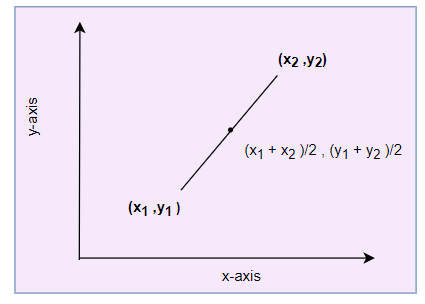# Midpoint Calculator for the Line segmentNote
• Enter the values of Coordinates of the two points
• Click on the calculate button.

Formula And concept used
$x_m = \frac {x_1 + x_2}{2}$
$y_m = \frac {y_1 + y_2}{2}$

### Midpoint Calculator

Example of Few questions where you can use this Midpoint Calculator
1. Find the Mid point of the line joining these two points.
i. A(2,3), B(4 ,5)
ii. A(-1,3), C(2,2)
iii. B(2 ,5) , C(1,7)
iv. A(1,1), C(9,5)